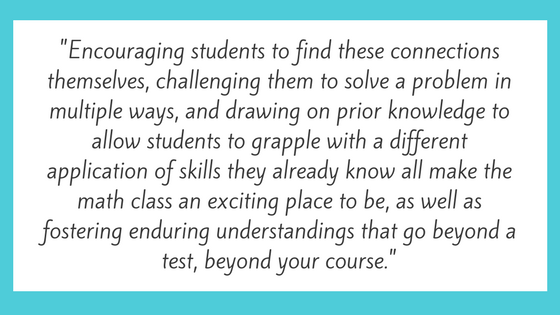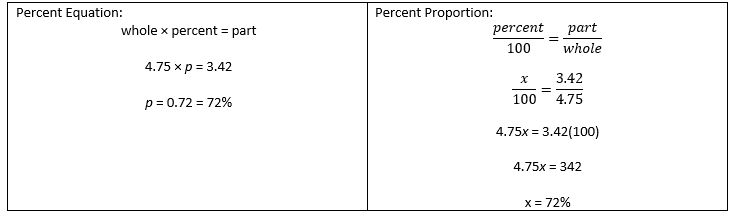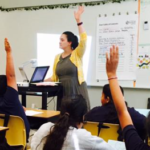# We Are All Math People: Conceptual Instruction in the Math Classroom

Anna Billa RheaEducator InsightsNow that we’ve all embraced our inner math nerd, we’re ready to launch into the why and how of teaching our children to be math nerds, too.

Math instruction has changed across most states in recent years. Another common refrain I hear from parents and teachers is:

“Why do we need the Common Core?”

“Why do/does my child/my students/I need to learn how to solve a multiplication problem in six different ways?”

If you have felt yourself thinking this, you are not alone. I believe this to be the response: these “new” standards are the best thing that could have happened to math education; learning multiple pathways, illustrating problems and answers in multiple ways – these are the skills that illuminate the connected web of mathematical understandings, that make math fun, and also make math make sense to those of us who are “just not math people.”

Recall my response to approaching math instruction after identifying the needs of my students outlined in last week’s blog post:
1. The goal is always for students to be able to recreate a process without the formula or rule, if they forget the rule.
2. In order to be able to recreate the learning, students have to have a flexible approach to problem solving. I have to illustrate for them how a proportion is a form of an equation or how a geometry problem is actually an algebra problem.

What does this look like? Consider the TNReady released item from 7th Grade Math:This is an example that could be seen in the real world. How often do we as adults work with percents? And yet, how often do we pause to think: is this right? …and maybe end up just looking it up online. In my original form of planning, I would have taught students to memorize two methods of solving: the percent equation and the percent proportion.When working with one method at a time, when all the problems looked the same, students would do quite well. But when the problem looked different, such as when the percent was over 100 percent, students would miss the problem more often than get it right. And, within a few weeks of new content, students would forget both rules, and have no idea where to start the problem.

So, how do I get my students to an enduring understanding? This begins with a deep understanding of concepts embedded within the math. In order to recreate the percent proportion or the percent equation if they have forgotten, students need to have a few core understandings that are founded in number sense – an understanding of how numbers and operations work together. When working with percents, students must know:

• A percent represents a part of a whole, when the whole has 100 parts. Connect to students’ understandings of fractions and decimals as partial values.
• A percent allows us to compare parts when the wholes have different amounts. In this way, a percent is inherently a proportional way of comparing values; we are comparing proportional parts.
• A percent that is less than 100% can be represented as a simple part of a whole. However, when the percent is greater than 100%, we have to consider the percent as a part of the original whole that gets added onto that whole.
• Percents will appear in a variety of ways; it is essential to consider what whole the percent is a part of, and work with that percent by adding or subtracting from the original value.
• Because a percent is out of 100, it is possible to work with percents as the part we have or the part we don’t have. If an item is 25% off, I can find the amount I would pay by finding 25% and subtracting it from the original price OR I can find 75% of the whole knowing that is the part I will pay.
• A percent operates within our base-ten number system. To find 10% of a number, I can treat the problem the same way I would as dividing by 10, using the trick of moving the decimal point one place to the left (10% of \$25.00 is \$2.50). I can use similar mental math tricks for other percents: 20% is double 10%; 15% is half of 10% added onto the 10%.

Side note: I always like to tell my students the story of  when I first started dating my husband, and I would show off by calculating tip without my phone. He had never learned this “trick” and would wait until I went to the restroom to calculate the tip himself on his phone. After I taught him this “trick,” we would have competitions to see who could do it faster: me in my head or him on his phone. I always beat the phone. Now, he never uses his phone to calculate tip.

This is not an exhaustive list of the concepts embedded within percents. At the end of the day, if my students understand what percents are, how they work, and what they represent, they will be able to recreate either method for solving a percent problem on paper or in the real world, OR solve the problem in pieces by applying one concept or understanding at a time. This is far more powerful than memorizing a strategy that they will forget by the next grade level.

Teaching these concepts is not an easy task. First, even the teachers who know math inside and out may not know all of the concepts embedded within the topic, especially as the concepts become more foundational. We may have learned those concepts at some point, but they become so automatic that at some point we stop realizing we’re doing them, and it is very difficult to bring ourselves back to that level.

Second, finding the right instructional tasks to develop student conceptual understanding is a major challenge. And, when we find the right tasks, how do we find the instructional time to spend on truly developing a concept when we have so many standards to teach?

There isn’t one answer to these questions, and it often requires a leap of faith that spending the concept-building time on the front end (and continuing to tie in throughout) will deepen understanding to the point that the rest of the content will be grasped more quickly.

Often, the answer to both building teacher and student understanding is similar. We go through parallel processes.

The foundation of almost all mathematics will lie within the same core concepts. As middle and high school teachers, or parents of middle and high school students, our tasks for understanding begin with elementary learning.

Revisit elementary standards. The Common Core is beautiful because it lays out multiple ways of developing concepts, including visuals. Know what multiplication and division are, what addition and subtraction are. Understand fact families and inverse operations. Know prime numbers, factors, and factorization. Know the distributive property, both with numbers and with variables – and how the distributive property involves pulling shared factors out of numbers. These principles will underlie almost everything we teach students in higher grades, and foundational gaps usually lie in one of these places.

Create visual models to illustrate these foundational concepts. Break numbers apart using a diagram, to show parts of a whole, or multiplication as groups with equal amounts in them. Look online to find manipulatives and other types of models, to see what other teachers have used. As a teacher, actually work through problems drawing the models yourself. Then, have your students use the models, too.

Introduce a concept through exploration and discovery before teaching the skill. Plan a lesson that allows students to develop the understanding themselves, generating the rule or deriving a formula based on foundational knowledge they already have.

Continuously draw back to the concept, even when solving skill-based problems. Require students to articulate in words why a skill works, through class discussion, partner discourse, or in written form. Or, require students to find an answer in three different ways, including a standard algorithm and a diagram or model. Ask students to solve a problem in different ways, then compare answers across a group.

Ask students to draw connections across content. When working with related concepts, ask students to name the skills they are using. Are they solving an algebraic equation when solving a proportion? Are they applying the distributive property when breaking a multi-digit multiplication problem down into easier problems to solve? Are they using place value to convert a decimal to a fraction, applying understanding of the base-ten number system? Almost every topic we teach in a math course connects to at least one other topic from the same course, as well as topics from previous courses. And almost every mathematical concept relates back to the same set of primary foundational understandings within the world of Math.

Create visual concept maps for students. Use classroom space wisely. Visually show students how concepts connect. Create a visual on the wall that represents equivalent values across columns: word form to expanded form to decimals to fractions to percents to diagrams. Create a web on the wall or on paper that represents how a set of subtopics derive from a central concept, as well as how the subtopics are connected. Make the classroom a living, breathing set of interconnected mathematical concepts.

The joy of math, the beauty of math, what makes math fun, is the connectedness of it. Topics connect because they are all related to a core set of foundational concepts that develop and play out in different ways. Encouraging students to find these connections themselves, challenging them to solve a problem in multiple ways, and drawing on prior knowledge to allow students to grapple with a different application of skills they already know all make the math class an exciting place to be, as well as fostering enduring understandings that go beyond a test, beyond your course.

Teachers and parents: we have the remarkable opportunity to explore mathematical concepts together with our students and children, creating a future generation of Math People.